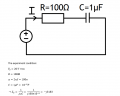#### Trang

Joined Jun 21, 2016
5Last week, I had had the pratical about how to use the Oscilloscope then I had the graph and the formula for Vr and Vc are:The question of profesor is why the resistor voltage lead the capacitor voltage by 90?
Thanks all guys for help!

#### MrChips

Joined Oct 2, 2009
27,675
Hmmm, you are missing some crucial information. What resistor? What capacitor?

You need to show a circuit diagram.
What is the value of the resistor and the value of the capacitor?
The phase angle is a function of frequency. What is the frequency?

How much calculus background do you have?
What is the derivative of a cosine function?

#### Trang

Joined Jun 21, 2016
5
Hmmm, you are missing some crucial information. What resistor? What capacitor?

You need to show a circuit diagram.
What is the value of the resistor and the value of the capacitor?
The phase angle is a function of frequency. What is the frequency?

How much calculus background do you have?
What is the derivative of a cosine function?
Really sorry for my shortcoming, here is all about my pratical#### shteii01

Joined Feb 19, 2010
4,644
The simple answer is that capacitor takes time to charge. Resistor is simply passing some current through, as soon as there is current flowing through the resistor, you have voltage across resistor. So voltage across resistor is establishing at the speed of current flowing through it, fast in other words. Capacitor does not work that way. Read the physics of the capacitor, it should be in a textbook that discusses physical composition of capacitor and the mechanics of capacitor operation.

#### Trang

Joined Jun 21, 2016
5
The simple answer is that capacitor takes time to charge. Resistor is simply passing some current through, as soon as there is current flowing through the resistor, you have voltage across resistor. So voltage across resistor is establishing at the speed of current flowing through it, fast in other words. Capacitor does not work that way. Read the physics of the capacitor, it should be in a textbook that discusses physical composition of capacitor and the mechanics of capacitor operation.

#### Trang

Joined Jun 21, 2016
5
And I'm sorry because of posting two same thread.
Because I thought that I shouldn't post this problem in this article which is only for Project so I tried to delete this thread but cannot find this button...

#### crutschow

Joined Mar 14, 2008
31,112
A capacitor requires charge be added to it before its voltage can change, a resistor doesn't.
So when you apply a voltage to the RC series combination, the resistor will have voltage across in instantly, but the capacitor voltage will slowly rise as it becomes charged from the current through the resistor.

The same thinks happens when the voltage drops and the capacitor starts to discharge. You now have to remove charge before the capacitor voltage can drop which means the capacitor voltage again lags the resistor voltage.

#### MrChips

Joined Oct 2, 2009
27,675
btw, the picture of the scope screen in post #1 does not match your question about 90° phase shift.
What is shown in the post #1 screen shot is 180° phase shift.

What gives?